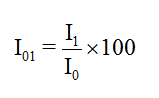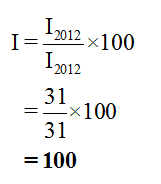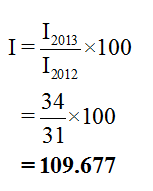# Between 2012 and 2013, the cost of a typical consumer’s market basket in Quarterville increased from 31 to 34.Assuming 2012 is the base year, calculate the index number for this market basket in 2012. Assuming the same base year, calculate the index number for this market basket in 2013.

Question
1 views

Between 2012 and 2013, the cost of a typical consumer’s market basket in Quarterville increased from 31 to 34.

Assuming 2012 is the base year, calculate the index number for this market basket in 2012.

Assuming the same base year, calculate the index number for this market basket in 2013.

check_circle

Step 1

Index number shows the change in a variable over time.

It can be calculated as follows:Here, I01 = Index number of year 1 taking year 0 as the base year.

I1 = Consumer basket in year 1

I0 = Consumer basket in year 0

Step 2

Now, 2012 is taken as the base year. So, the index number for market basket in 2012 can be calculated as:

I2012 = Consumer basket in 2012Index number in 2013 taking 2012 as the base year can be calculated as:

I2013 = Consumer basket in 2013...

### Want to see the full answer?

See Solution

#### Want to see this answer and more?

Solutions are written by subject experts who are available 24/7. Questions are typically answered within 1 hour.*

See Solution
*Response times may vary by subject and question.
Tagged in